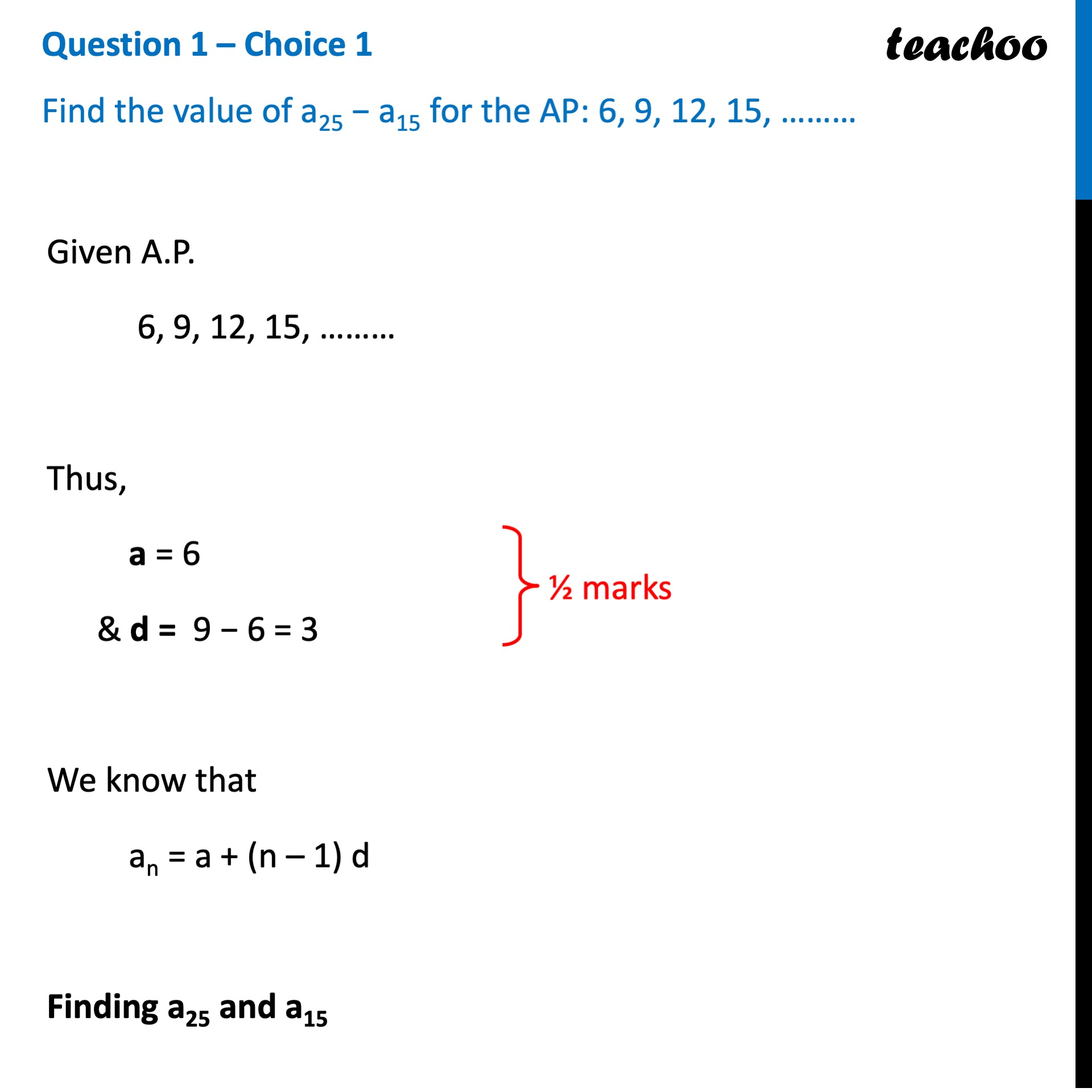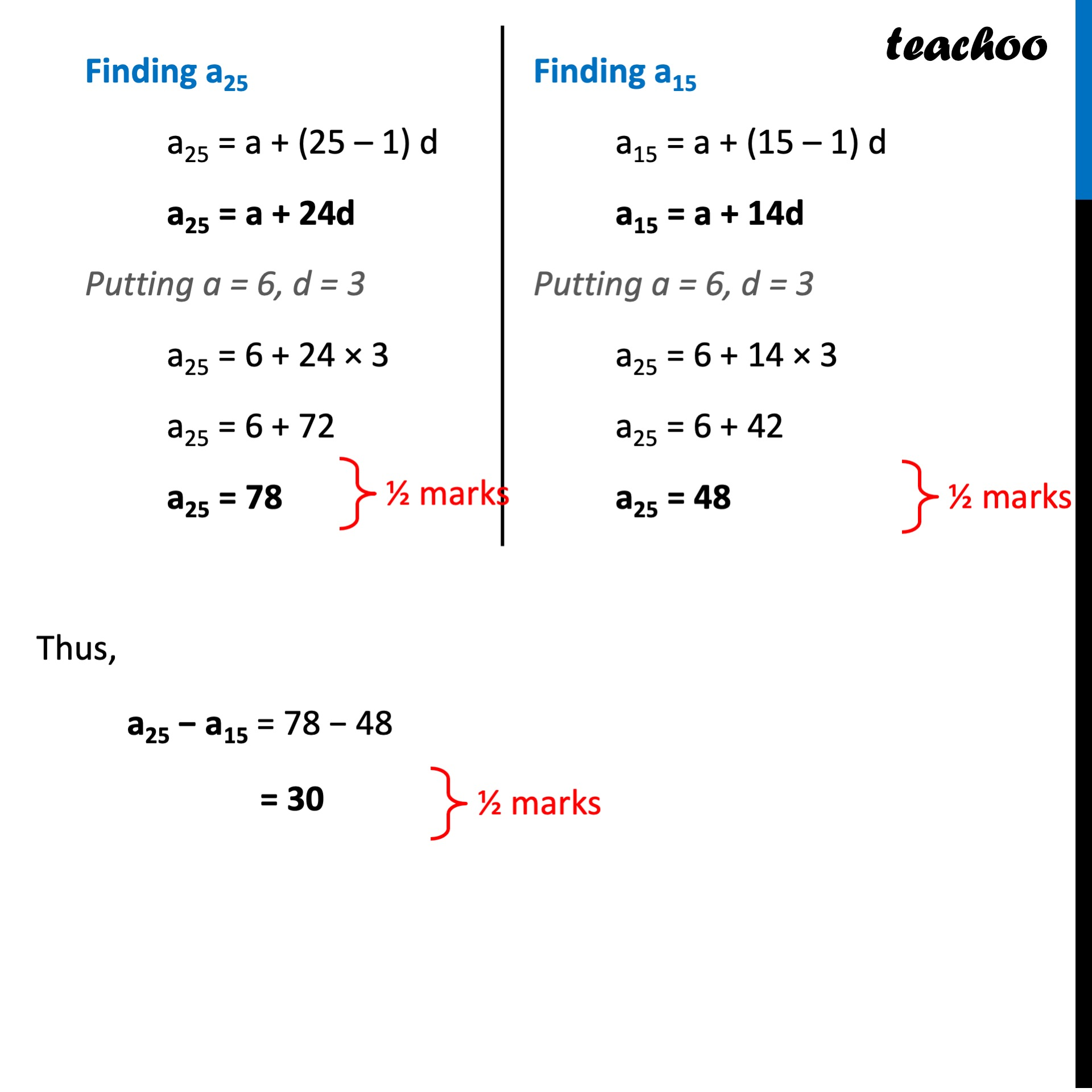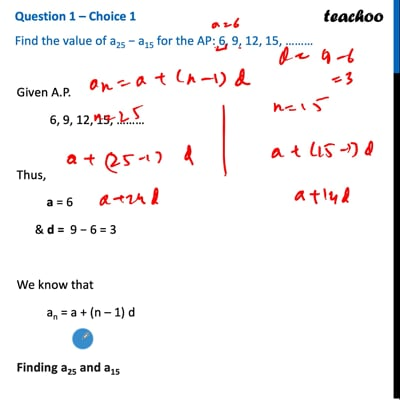CBSE Class 10 Sample Paper for 2022 Boards - Maths Standard [Term 2]

Class 10
Solutions of Sample Papers for Class 10 Boards

## Find the value of a 25 - a 15 for the A.P: 6,9,12,15,...........

This question is similar to Question 8 or 2nd CBSE Class 10 Sample Paper 2019 BoardsThis video is only available for Teachoo black users

Introducing your new favourite teacher - Teachoo Black, at only ₹83 per month

### Transcript

Question 1 – Choice 1 Find the value of a25 − a15 for the AP: 6, 9, 12, 15, ……… Given A.P. 6, 9, 12, 15, ……… Thus, a = 6 & d = 9 − 6 = 3 We know that an = a + (n – 1) d Finding a25 and a15 Finding a25 a25 = a + (25 – 1) d a25 = a + 24d Putting a = 6, d = 3 a25 = 6 + 24 × 3 a25 = 6 + 72 a25 = 78 Finding a15 a15 = a + (15 – 1) d a15 = a + 14d Putting a = 6, d = 3 a25 = 6 + 14 × 3 a25 = 6 + 42 a25 = 48 Thus, a25 − a15 = 78 − 48 = 30